# Calculate a rolling sum in the Data Pipeline

Data Pipeline

You can calculate a moving average in Chartio by using our Moving Average preset function in the Data Pipeline using an Add Column or Edit Column Pipeline step. In the event where you’d like to calculate a rolling sum (or moving sum), you can easily edit the Moving Average to multiply it by the selected period.

In the example below, we’re creating a chart that pulls the count of users per day. Here, we also want to include a column that calculates the rolling sum of the count of users in the last 7 days. This is different from a standard running total because it only includes the sum of the previous 7 rows of data.

To do this, follow the steps below:

1. Start by building a chart for the count of users per day.

Here, we’re using our Chartio Demo Data Source and pulling the Count of distinct User Id as a measure and the Day of Created Date as a dimension, both columns from our Users table.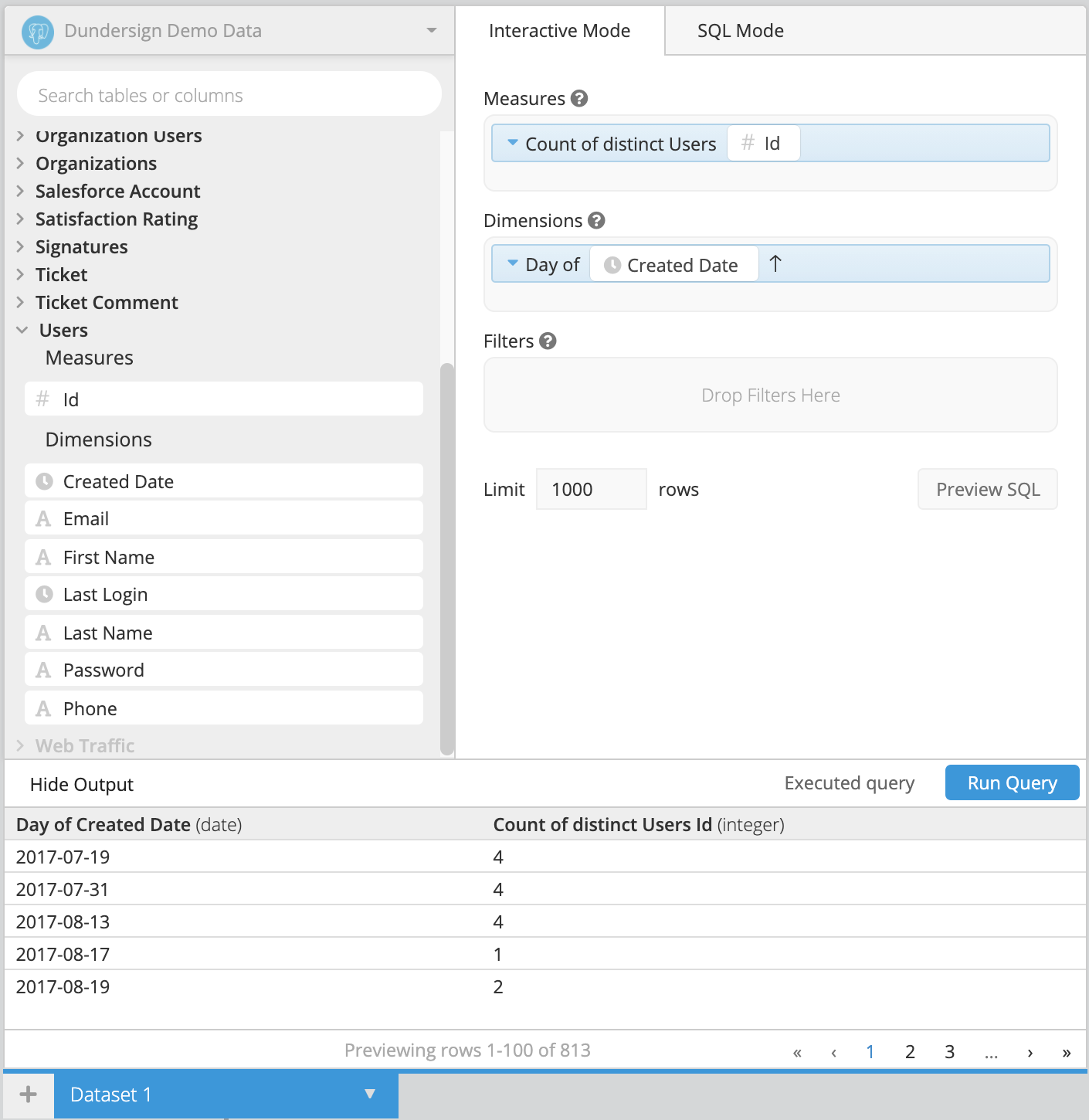2. Add a new column in the Data Pipeline to calculate the moving average.

Select +Add Transformation below your Dataset query and choose Add Column from the options. Then, select Moving average from the Formula Type dropdown and choose the “Count of distinct Users User Id” column as the Input Column. You’ll finally want to enter “7” in the Trailing Rows input box to only calculate the moving average for the last 7 days. Click Apply & Close when done.

Note: If you don’t have rows for every day and want to make sure you calculate null values for the last week, add a Zero Fill Data Pipeline step before the Add Column step.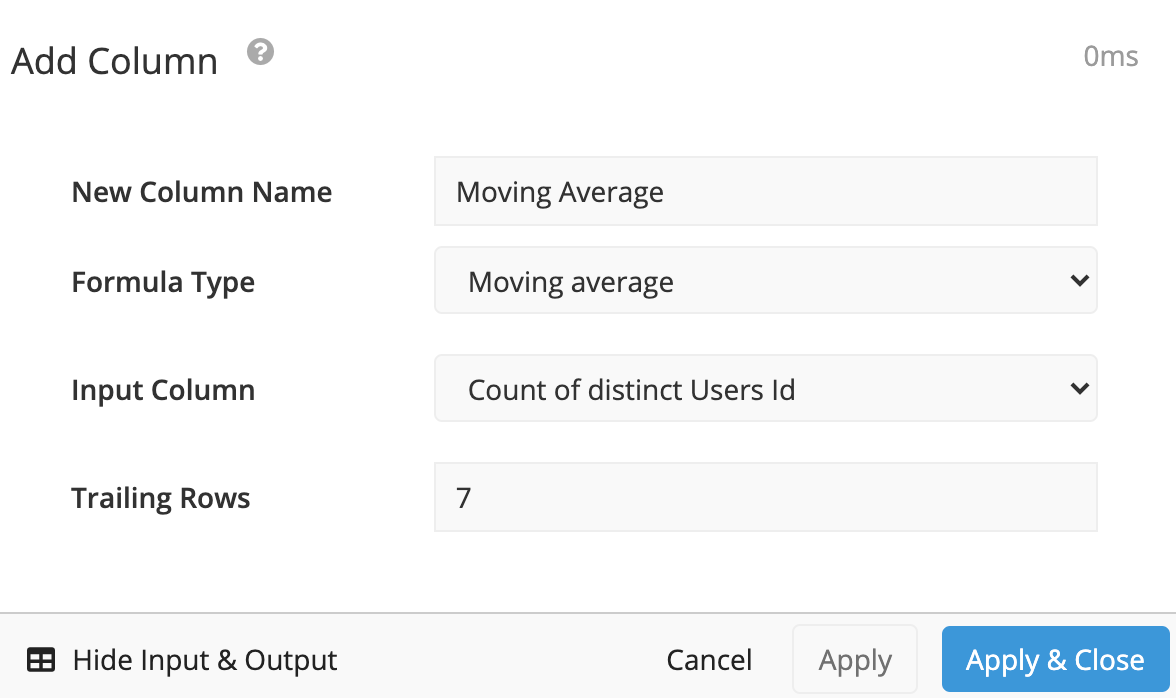3. The moving average is simply the sum of the last 7 values divided by 7. Therefore, we can get our rolling sum by multiplying the moving average by seven.

Add a new column to do exactly that. Simply select Custom formula from the Formula Type dropdown and enter the following into the Formula field, replacing Moving average with your custom column from step 2.

``````"Moving average" * 7
``````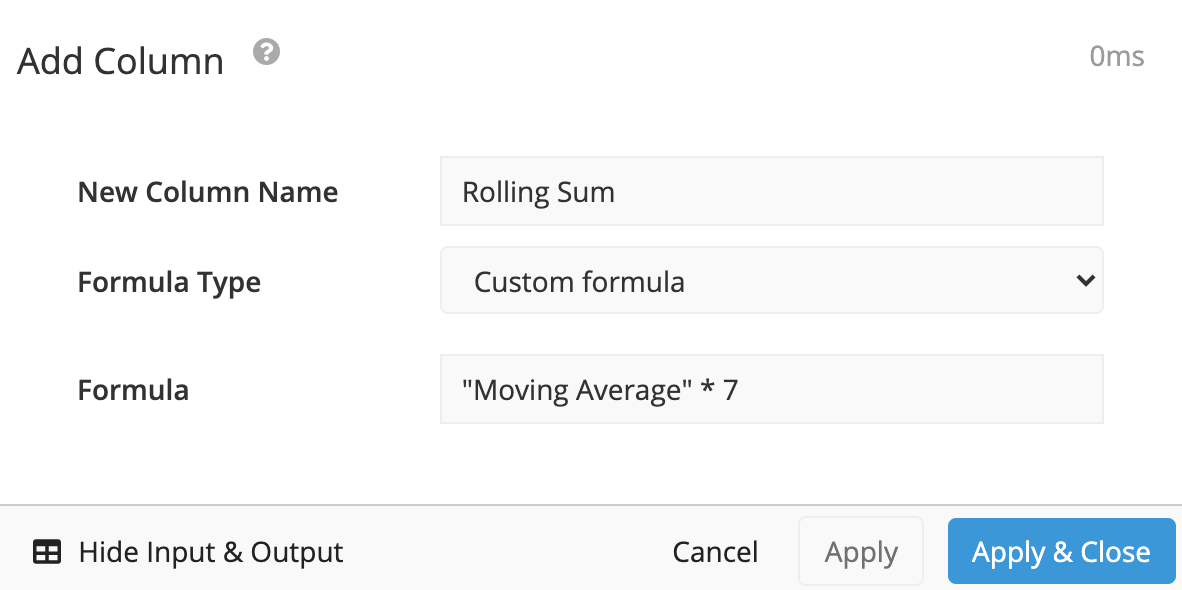4. Add a Hide Columns step in the Pipeline to hide your Moving average column. Click Apply & Close.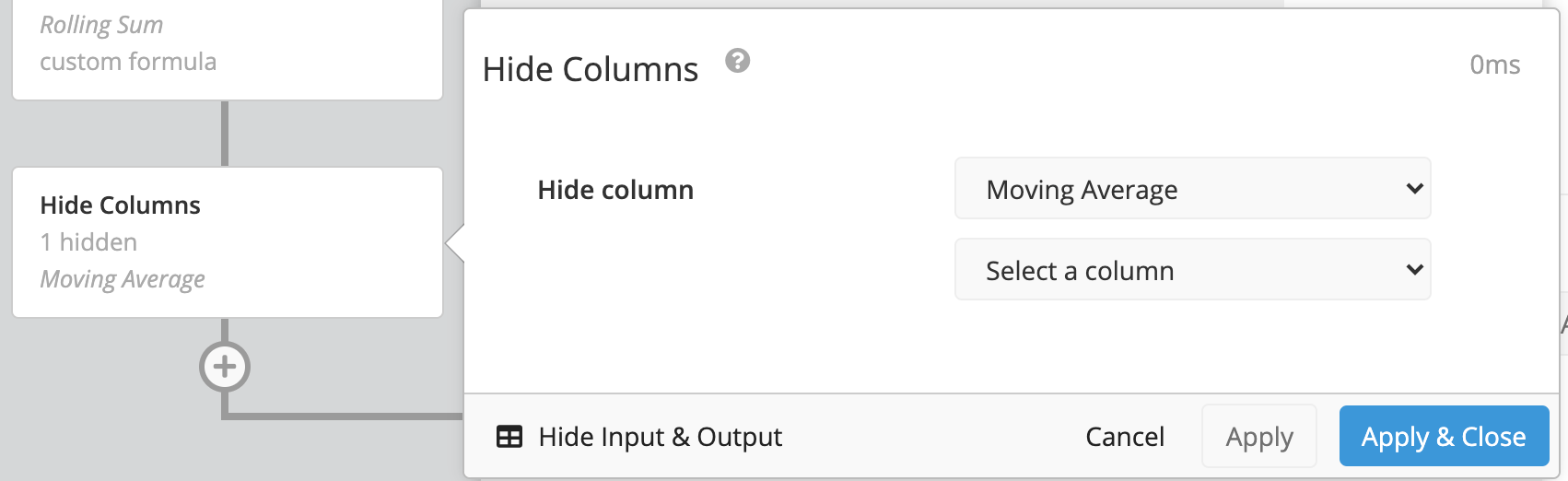5. Switch your chart to a Line chart from the chart types to visualize your daily user count compared to the rolling user sum. Don’t forget to save your chart to your dashboard when done.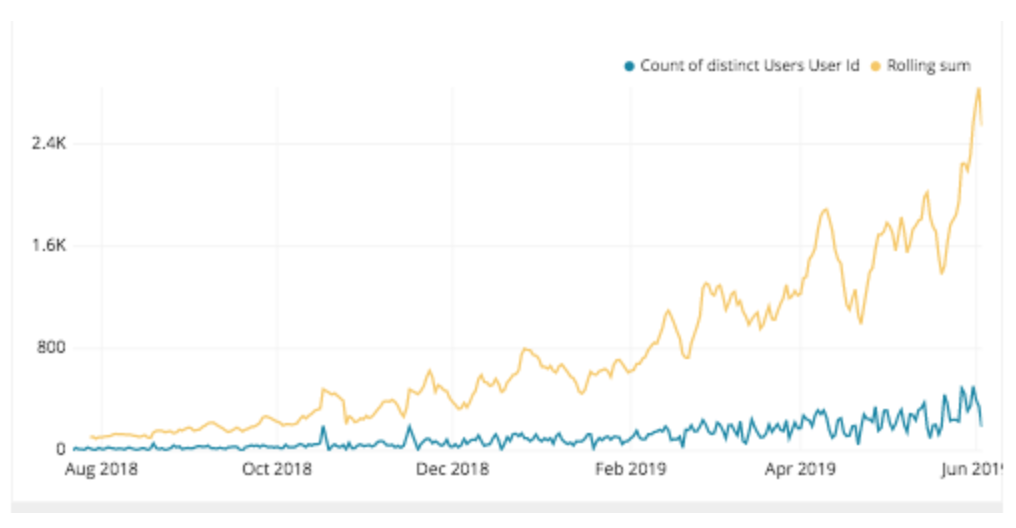See more